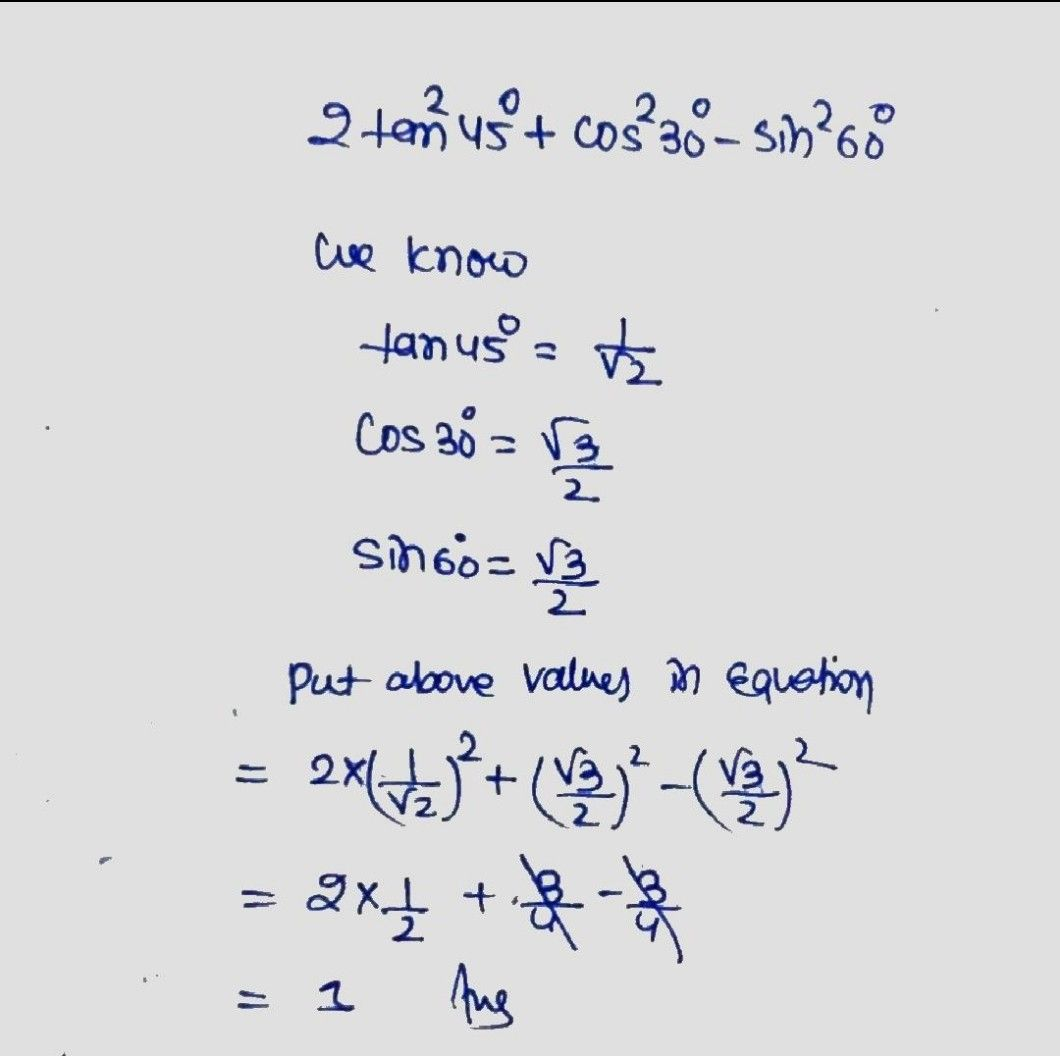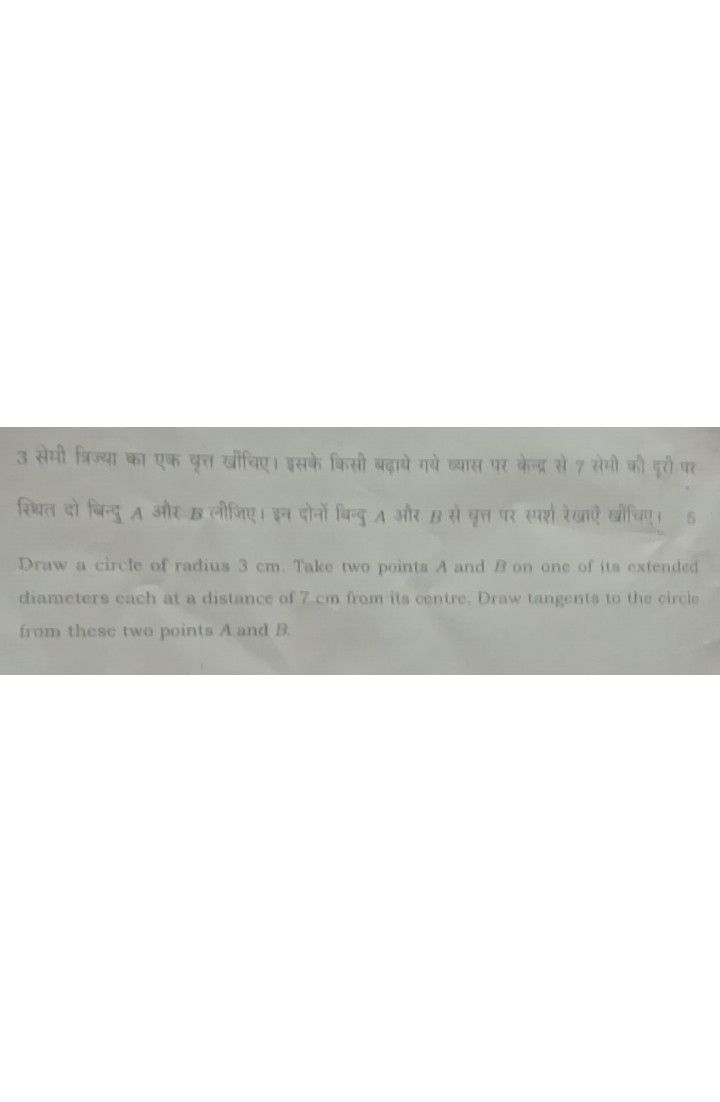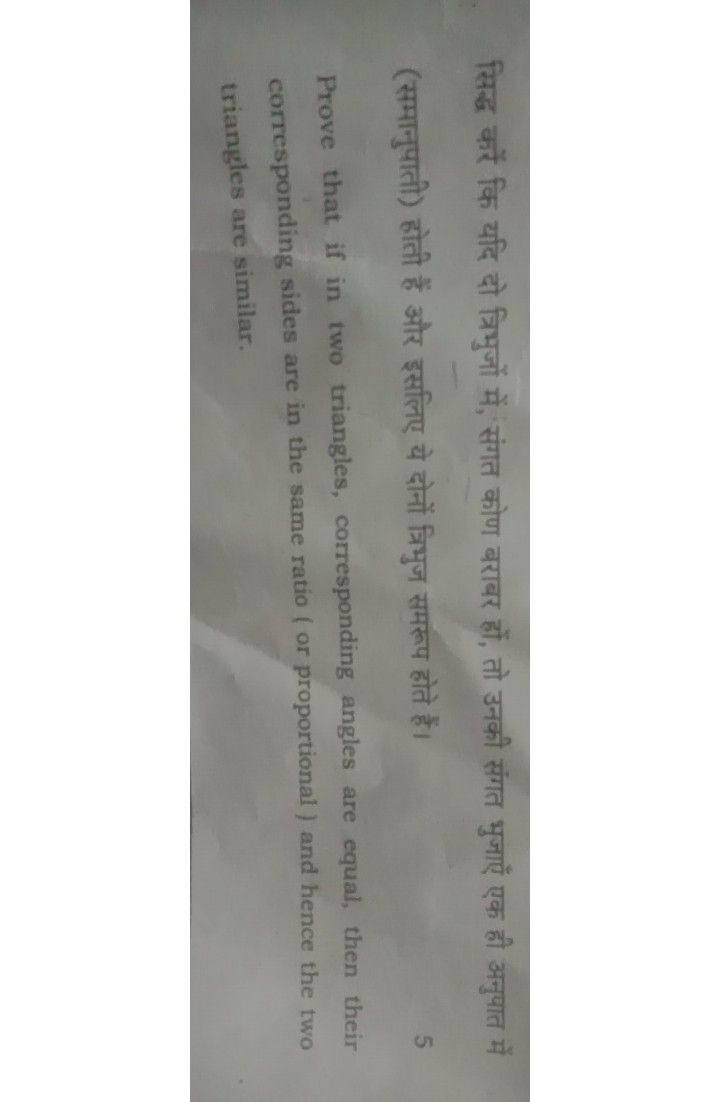Symbol
ProblemHA ana $2tan^{2}45^{°}+cos^{2}30^{°}-sin^{2}60^{°}$ Evaluate : $2tan^{2}45^{°}+cos^{2}30^{°}-sin^{2}60^{°}$